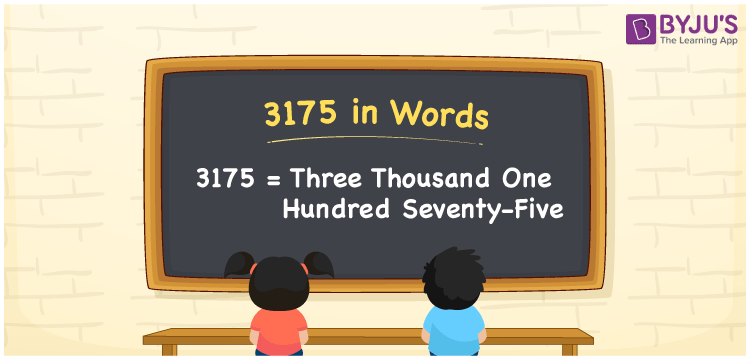# 3175 in Words

The numeral 3175 in words “three thousand one hundred seventy-five”. The number 3175 is a natural number and it has four-digits. Also, 3175 is a cardinal number. For example, the cost of 3 chairs is Rs. 3175. Now, let us discuss how to spell the number 3175 in words using the place value system.

 3175 in Words: Three Thousand One Hundred Seventy-five. Three Thousand One Hundred Seventy-five in Numerical Form: 3175.

## 3175 in English Words## How to Write 3175 in Words?

The below table represents the place values of the number 3175.

 Thousands Hundreds Tens Ones 3 1 7 5

The expanded form of 3175 is as follows:

= 3 × Thousand + 1 × Hundred + 7 × Ten + 5 × One

= 3 × 1000 + 1 × 100 + 7 × 10 + 5 × 1

= 3000 + 100 + 70 + 5

= 3175

= Three thousand one hundred seventy-five

Hence, 3175 in words is three thousand one hundred seventy-five.

3175 in words – Three thousand one hundred seventy-five

Is 3175 an odd number? – Yes

Is 3175 an even number? – No

Is 3175 a perfect square number? – No

Is 3175 a perfect cube number? – No

Is 3175 a prime number? – No

Is 3175 a composite number? – Yes

## Frequently Asked Questions on 3175 in Words

Q1

### Write 3175 in words.

3175 in words is three thousand one hundred seventy-five.

Q2

### Simplify 3100 + 75, and express it in words.

Simplifying 3100 + 75, we get 3175. Hence, 3175 in words is three thousand one hundred seventy-five.

Q3

### Is 3175 an odd number?

Yes, 3175 is an odd number.